# Concentration

How many grams of 65% and 50% acid is to be mixed to give 240 g acid with a concentration of 60%?

a =  160
b =  80

### Step-by-step explanation:

a+b= 240
0.65a+0.50b = 0.60•240

a+b= 240
0.65•a+0.50•b = 0.60•240

a+b = 240
0.65a+0.5b = 144

a = 160
b = 80

Our linear equations calculator calculates it.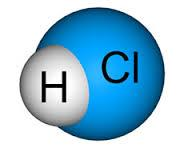Did you find an error or inaccuracy? Feel free to write us. Thank you!Tips to related online calculators
Do you have a linear equation or system of equations and looking for its solution? Or do you have a quadratic equation?

## Related math problems and questions:

• Acid solutionBy adding 250 grams of a 96% sulfuric acid solution to its 3% solution, we changed its initial concentration to 25%. How many grams of 3% of the acid was used for dilution?
• Alcohol mixingHow much 55% alcohol we must pour into 1500 g 80% alcohol to form a 60% alcohol? How much 60% alcohol created?
• SolutionsHow much 60% solution and how much 35% solution is needed to create 100 l of 40% solution?
• Alcohol solutionsWe have to produce 2 liters of 60% alcohol from 55% and 80%. How many of which ones will we use in the solution?
• GramsHow many grams of 5% solution do we need to add to 100g of 50% solution to get a 20% solution?
• Alcohol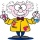6l 48% alcohol, how much 52% alcohol must be added to give 50% alcohol?
• Alcohol mixtureFrom 55% and 80% alcohol, we have to produce 0.2 kg of 60% alcohol. How many of them do we use in the solution?
• Hydrochloric acidDetermine the concentration of which must have a solution of hydrochloric acid that mixing 10 l of the solution with 8 liters of 26% solution to get the solution with a concentration of 50%?
• Acid evaporationHow many kilograms of water do we have to evaporate from 100 kg of 32% acid to make it 80% concentration?
• SpiritFrom 55% and 80% spirit we would like to produce 0.2 kg of 60% spirit. How many of them we must use in a solution?
• Copper sulphate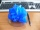How much g of water do we have to add to 240 g of an 84% CuSO4 solution to produce a 60% solution? (Express the mass of crystalline CuSO4 in the original solution and in the resulting solution and compare them. )
• AlcoholHow many 55% alcohol we need to pour into 14 liters 75% alcohol to get p3% alcohol? How many 65% alcohol we get?
• Solutions, mixturesHow many liters of 70% solution we must add to 5 liters of 30% solution to give us a 60% solution?
• Pouring alcohol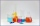100 liters of alcohol has 70% How many liters of water need to be added to have 60% alcohol?
• CuSO4 mixtureHow many grams of solid CuSO4 we have to add to 450g of 15% CuSO4 solution to produce a 25% solution?
• Acids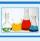70% acid made from the same acid of two different concentrations. Amount weaker acid to the stronger acid is in ratio 2:1. What was the concentration of the weaker acid if stronger had 91% concentration?
• SolutionWhat amount of 80% solution and what amount of water is needed to mix in order to obtain two liters of 60% solution of this mixture?